﻿ Design of Normal Mode Helical Antenna in SAW Temperature Sensor Temperature Measurement System_

China sensor manufacturers

# Design of Normal Mode Helical Antenna in SAW Temperature Sensor Temperature Measurement System

1.1 Model design
(1) Parameter calculation: The central operating frequency fc of the system is 915 MHz, and the calculation formula of the central working wavelength λc is: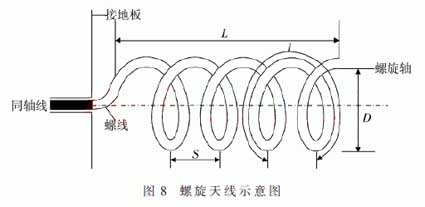The characteristics of the helical antenna are determined by πD < λ. In this design, πD < 0.5 λ, and therefore it is a normal mode helical antenna.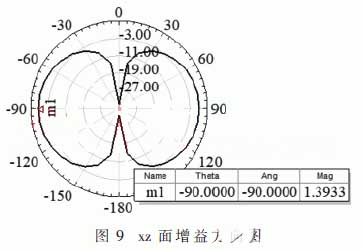(2) Setting of boundary conditions: When HFSS analyzes an antenna, it is first necessary to set the radiation boundary, and the distance between the radiating surface and the radiator must be greater than a quarter of the operating wavelength. According to the structure of the normal mode helical antenna, a cuboid model is created with vertex coordinates of (-90, -90, -90), each axis is 180 mm long, and the material is air, named AirBox. After the cuboid model is built, its surface needs to be set to the radiation boundary condition.

(3) Setting of the incentive port: There are many excitation methods in HFSS. Since the input port of the design mode antenna is inside the model, the Lumped Port is used and the port impedance is set to 50 Ω.

(4) Solving settings: Set the solution frequency to 915 MHz, the maximum number of iterations for meshing is 15 and the convergence error is generally 0.02. The sweep range is set from 0.5 GHz to 1.5 GHz in steps of 0.01 GHz in the Linear Step.

1.2 Simulation results and analysis
According to the above parameter calculation and setting, the HFSS model is established. The normal mode spiral antenna is mainly composed of a spiral coil, a grounding plate and a PCB board. In this design, the number of turns of the spiral coil is set to 9.5 turns, and the pitch is set to 2 mm; The size of the grounding plate is 27 mm × 30 mm; The excitation method is lumped port excitation; the PCB board volume is 30 mm × 30 mm × 1 mm.

The simulation results show that the normal mode helical antenna has a voltage standing wave ratio of about 1.48 and less than 1.5 at the center operating frequency of 915 MHz, indicating that the matching is good and the transmission characteristics are good, which achieves the goal of this design.

The xz plane gain pattern of the normal mode helical antenna is shown in Fig 9. At this time, the maximum radiation direction of the antenna is in a plane perpendicular to the spiral axis, and the pattern in any one plane including its axis is a figure-eight shape. In the figure, the m1 point is the maximum gain point. It can be seen that the maximum gain of this design is about 1.39 dB, which is greater than 0, which achieves the design goal of the helical antenna.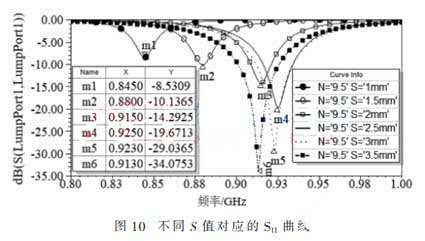1,3 optimized design
(1) Influence of pitch on working frequency
The pitch of the normal mode helical antenna is represented by the variable S, which can be concluded from Figure 10: Keep the other dimensions unchanged, change the pitch S, the center working frequency of the normal spiral antenna will change with the increase of S, first increase and then decrease. Therefore, in the design, we must first find the inflection point. Before the inflection point, the center working frequency should increase with the increase of S. After the inflection point, the center operating frequency is reduced as S increases, and then narrowed down according to the design goals.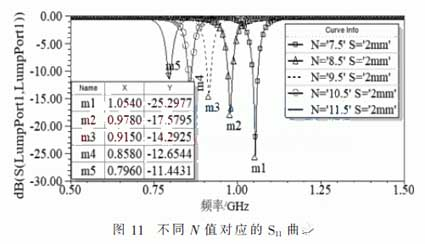(2) The effect of the number of turns on the operating frequency
The number of turns of the normal mode helical antenna is represented by a variable N. It can be seen from Fig. 11 that when the N values are 7.5 mm, 8.5 mm, 9.5 mm, 10.5 mm, and 11.5 mm, the corresponding resonant frequencies are 1 054 MHz, 978 MHz, 915 MHz, 858 MHz, and 796 MHz, respectively. It can be seen from the simulation results that the increase of the number of turns N causes the central operating frequency of the normal mode helical antenna to gradually decrease while keeping the other dimensions constant.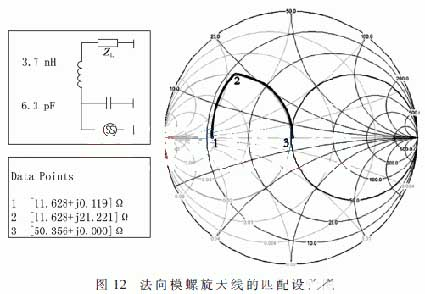(3) Matching of normal mode helical antenna
For the normal mode helical antenna, the Smith v2.0 software is used for impedance matching in this design. The essence is to use the Smith chart principle for impedance matching. Figure 12 shows the matching of the normal helical antenna with Smith v2.0 software. The set frequency is 915 MHz and the initial input impedance is (11.628+j0.119) Ω. Obtain its position in the Smith chart as point 1. After connecting an inductor in series, connect a capacitor in parallel and the impedance can reach 50 Ω. In the Smith chart, point 1 is rotated clockwise to point 2, then clockwise to point 3 to complete the match. In the case of physical production, the values of the inductance and capacitance also need to be adjusted according to the actual situation.

4 Conclusion
This paper mainly designs two antennas for transmitting and receiving in the passive wireless SAW temperature sensor temperature measurement system. According to the different division of the transmitting antenna and the receiving antenna, combined with the application scenario, the reader antenna is selected as a PIFA antenna, and the sensor antenna is designed as a normal mode helical antenna. By establishing a model and optimizing the design and matching design, the design goal was finally achieved. The simulation results show that the resonant frequencies of the two antennas are all 915 MHz, the standing wave ratio is less than 1.5, and the input impedance is about 50 Ω, which achieves matching. The maximum gain of the PIFA antenna is 3.34 dB, and the gain of the normal mode helical antenna is 1.39 dB, which meets the parameters in the design.

﻿
﻿